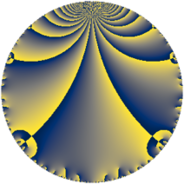# Properties

 Label 165.4.aLevel $165$ Weight $4$ Character orbit 165.a Rep. character $\chi_{165}(1,\cdot)$ Character field $\Q$ Dimension $20$ Newform subspaces $8$ Sturm bound $96$ Trace bound $4$

# Related objects

## Defining parameters

 Level: $$N$$ $$=$$ $$165 = 3 \cdot 5 \cdot 11$$ Weight: $$k$$ $$=$$ $$4$$ Character orbit: $$[\chi]$$ $$=$$ 165.a (trivial) Character field: $$\Q$$ Newform subspaces: $$8$$ Sturm bound: $$96$$ Trace bound: $$4$$ Distinguishing $$T_p$$: $$2$$

## Dimensions

The following table gives the dimensions of various subspaces of $$M_{4}(\Gamma_0(165))$$.

Total New Old
Modular forms 76 20 56
Cusp forms 68 20 48
Eisenstein series 8 0 8

The following table gives the dimensions of the cuspidal new subspaces with specified eigenvalues for the Atkin-Lehner operators and the Fricke involution.

$$3$$$$5$$$$11$$FrickeDim.
$$+$$$$+$$$$+$$$$+$$$$1$$
$$+$$$$+$$$$-$$$$-$$$$3$$
$$+$$$$-$$$$+$$$$-$$$$3$$
$$+$$$$-$$$$-$$$$+$$$$3$$
$$-$$$$+$$$$+$$$$-$$$$2$$
$$-$$$$+$$$$-$$$$+$$$$4$$
$$-$$$$-$$$$+$$$$+$$$$4$$
Plus space$$+$$$$12$$
Minus space$$-$$$$8$$

## Trace form

 $$20 q + 68 q^{4} + 12 q^{6} + 64 q^{7} + 180 q^{9} + O(q^{10})$$ $$20 q + 68 q^{4} + 12 q^{6} + 64 q^{7} + 180 q^{9} + 60 q^{10} + 48 q^{12} - 56 q^{13} - 104 q^{14} - 60 q^{15} + 300 q^{16} - 128 q^{17} + 152 q^{19} + 40 q^{20} + 264 q^{21} - 88 q^{22} - 136 q^{23} + 324 q^{24} + 500 q^{25} - 896 q^{26} - 24 q^{28} - 64 q^{29} + 192 q^{31} - 280 q^{32} - 132 q^{33} + 352 q^{34} - 80 q^{35} + 612 q^{36} + 504 q^{37} + 104 q^{38} + 336 q^{39} + 420 q^{40} - 656 q^{41} - 384 q^{42} - 784 q^{43} + 616 q^{44} + 1264 q^{46} + 472 q^{47} + 576 q^{48} - 28 q^{49} + 504 q^{51} - 32 q^{52} - 80 q^{53} + 108 q^{54} - 440 q^{55} - 408 q^{56} - 168 q^{57} - 2088 q^{58} - 736 q^{59} + 180 q^{60} + 696 q^{61} - 5128 q^{62} + 576 q^{63} - 1292 q^{64} - 320 q^{65} - 1136 q^{68} - 408 q^{69} + 680 q^{70} - 936 q^{71} + 3016 q^{73} - 4624 q^{74} + 544 q^{76} + 352 q^{77} + 432 q^{78} + 88 q^{79} - 1440 q^{80} + 1620 q^{81} + 4216 q^{82} - 2176 q^{83} + 2784 q^{84} + 1360 q^{85} + 2928 q^{86} - 864 q^{87} - 1056 q^{88} - 952 q^{89} + 540 q^{90} + 736 q^{91} - 6728 q^{92} + 3456 q^{93} - 6960 q^{94} - 1040 q^{95} + 2244 q^{96} + 1336 q^{97} + 2264 q^{98} + O(q^{100})$$

## Decomposition of $$S_{4}^{\mathrm{new}}(\Gamma_0(165))$$ into newform subspaces

Label Dim $A$ Field CM Traces A-L signs $q$-expansion
$a_{2}$ $a_{3}$ $a_{5}$ $a_{7}$ 3 5 11
165.4.a.a $1$ $9.735$ $$\Q$$ None $$0$$ $$-3$$ $$-5$$ $$2$$ $+$ $+$ $+$ $$q-3q^{3}-8q^{4}-5q^{5}+2q^{7}+9q^{9}+\cdots$$
165.4.a.b $1$ $9.735$ $$\Q$$ None $$1$$ $$3$$ $$-5$$ $$36$$ $-$ $+$ $-$ $$q+q^{2}+3q^{3}-7q^{4}-5q^{5}+3q^{6}+\cdots$$
165.4.a.c $2$ $9.735$ $$\Q(\sqrt{17})$$ None $$-1$$ $$6$$ $$-10$$ $$-4$$ $-$ $+$ $+$ $$q-\beta q^{2}+3q^{3}+(-4+\beta )q^{4}-5q^{5}+\cdots$$
165.4.a.d $3$ $9.735$ 3.3.23612.1 None $$-4$$ $$-9$$ $$-15$$ $$-4$$ $+$ $+$ $-$ $$q+(-1-\beta _{1})q^{2}-3q^{3}+(7+\beta _{1}+\beta _{2})q^{4}+\cdots$$
165.4.a.e $3$ $9.735$ 3.3.47528.1 None $$-2$$ $$9$$ $$-15$$ $$10$$ $-$ $+$ $-$ $$q+(-1+\beta _{1})q^{2}+3q^{3}+(10+\beta _{2})q^{4}+\cdots$$
165.4.a.f $3$ $9.735$ 3.3.788.1 None $$1$$ $$-9$$ $$15$$ $$-16$$ $+$ $-$ $+$ $$q+\beta _{2}q^{2}-3q^{3}+(-2-\beta _{1})q^{4}+5q^{5}+\cdots$$
165.4.a.g $3$ $9.735$ 3.3.1957.1 None $$1$$ $$-9$$ $$15$$ $$6$$ $+$ $-$ $-$ $$q-\beta _{1}q^{2}-3q^{3}+(6+\beta _{1}+2\beta _{2})q^{4}+\cdots$$
165.4.a.h $4$ $9.735$ 4.4.1540841.1 None $$4$$ $$12$$ $$20$$ $$34$$ $-$ $-$ $+$ $$q+(1-\beta _{1})q^{2}+3q^{3}+(7-\beta _{1}+\beta _{3})q^{4}+\cdots$$

## Decomposition of $$S_{4}^{\mathrm{old}}(\Gamma_0(165))$$ into lower level spaces

$$S_{4}^{\mathrm{old}}(\Gamma_0(165)) \cong$$ $$S_{4}^{\mathrm{new}}(\Gamma_0(5))$$$$^{\oplus 4}$$$$\oplus$$$$S_{4}^{\mathrm{new}}(\Gamma_0(11))$$$$^{\oplus 4}$$$$\oplus$$$$S_{4}^{\mathrm{new}}(\Gamma_0(15))$$$$^{\oplus 2}$$$$\oplus$$$$S_{4}^{\mathrm{new}}(\Gamma_0(33))$$$$^{\oplus 2}$$$$\oplus$$$$S_{4}^{\mathrm{new}}(\Gamma_0(55))$$$$^{\oplus 2}$$